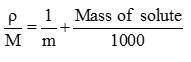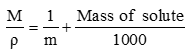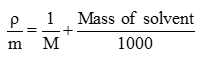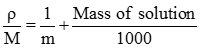# Topic: Chemistry Solutions (Test 1)

Topic: Chemistry Solutions
Q.1
Which among the following is miscible in each other?
A. Methanol and water
B. All of these
C. Methanol and benzene
D. Benzene and water
Explaination / Solution:

Methanol and Water both are Polar. There is intermolecular Hydrogen Bonding interaction.

Workspace
Report
Q.2
Which among the following is an example of liquid in solid?
A. Hydrated salts
B. Alloys
C. Sugar solution
D. Aerated drinks
Explaination / Solution:

Hydrated salts have water molecule of hydration as solute.

Workspace
Report
Q.3
Which among the following is an example of a solid solution in which the solute is a gas?
A. Pumice stone
B. Hydrated salts
C. Aerated drinks
D. Humidity in air
Explaination / Solution:

Pumice stone is example of gas in Solid.

Workspace
Report
Q.4
The type of intermolecular interaction present in n-Hexane and n-Octane is
A. Ion-dipole interaction
B. Dipole-dipole interaction
C. Vander wall forces of interaction
D. Hydrogen bonding
Explaination / Solution:

Both are Non Polar.

Workspace
Report
Q.5
Mathematical expression relating molarity and molality is
A.B.C.D.Explaination / Solution:

m= moles of solute / mass of solvent. M= moles of solute / volume of solution.

Workspace
Report
Q.6
The tanks used by divers are filled with air diluted with
A. Argon
B. Helium
C. Hydrogen
D. Nitrogen
Explaination / Solution:

Size of Helium is small so does not causes “Bents” to divers when the dive back to surface.

Workspace
Report
Q.7
Greater the value of KH, lower will be the
A. Pressure
B. Concentration
C. Solubility
D. Temperature
Explaination / Solution:

Pgas= KH× Xgas.Xgas
is measure of solubility of gas.
Workspace
Report
Q.8
Which among the following is soluble in water?
A. Phenol
B. Benzene
C. Formic acid
D. Chloroform
Explaination / Solution:

Because of hydrogen bonding.

Workspace
Report
Q.9
Which among the following is soluble in n-octane?
A. Methanol
B. Hexane
C. Chloroform
D. Ethylene glycol
Explaination / Solution:

Like dissolves in like

Workspace
Report
Q.10
The use of pressure cooker reduces cooking time because it creates
A. High pressure
B. Low pressure
C. Low temperature
D. High temperature